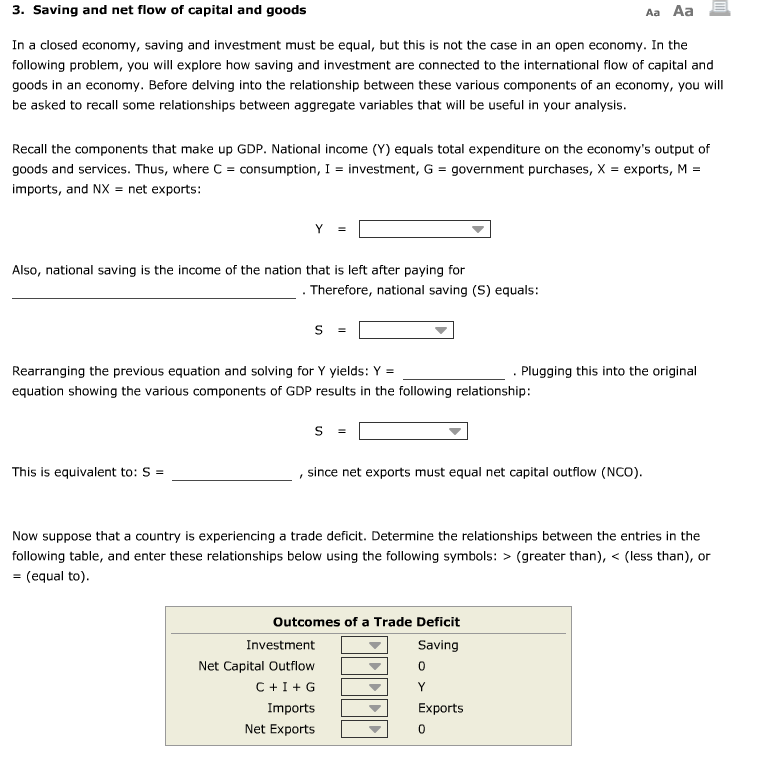# Suppose that a country is experiencing a trade deficit

\$1.00Text: In a closed economy, saving and investment must be equal, but this is not the case in an open economy. In the following problem, you will explore how saving and investment are connected to the international flow of capital and goods in an economy. Before delving into the relationship between these various components of an economy, you will be asked to recall some relationships between aggregate variables that will be useful in your analysis. Recall the components that make up GDP. National income (Y) equals total expenditure on the economy’s output of goods and services. Thus, where C = consumption, I = investment, G = government purchases, X = exports, M = imports, and NX = net exports: Also, national saving is the income of the nation that is left after paying for . Therefore, national saving (S) equals: Rearranging the previous equation and solving for Y yields: Y = . Plugging this into the original equation showing the various components of GDP results in the following relationship: This is equivalent to: S = , since net exports must equal net capital outflow (NCO). Now suppose that a country is experiencing a trade deficit. Determine the relationships between the entries in the following table, and enter these relationships below using the following symbols: > (greater than), < (less than), or = (equal to).

SKU: economics-problem Category: